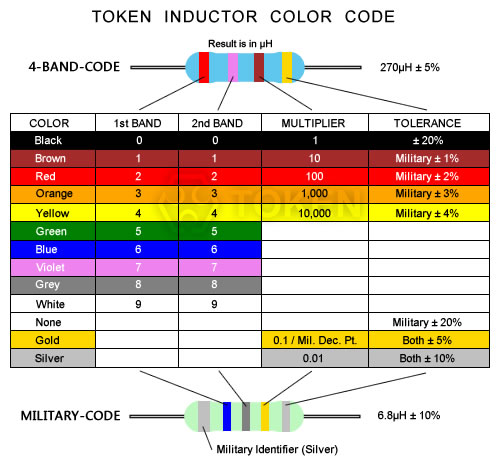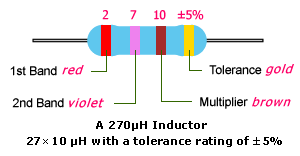# Inductor Color Code

## How To Read The Inductor Color Codes

### EIA Standard & Military Standard##### EIA Standard & Military Standard##### Inductor Color Code - Ex. 270μH ± 5%
1. First find the tolerance band, it will typically be gold (5%) and sometimes silver (10%).
2. Starting from the other end, identify the first band - write down the number associated with that color; in this case Red is 2.
3. Now 'read' the next color, here it is Violet so write down a 7 next to the two. (you should have '27' so far.)
4. Now read the third or 'multiplier' band and write down that number of 10.
5. In this example, the 'multiplier' band is Brown so we get 270 μH.
6. If the 'multiplier' band is Gold move the decimal point one to the left.
7. If the 'multiplier' band is Silver move the decimal point two places to the left.

### Inductance Tolerance Codes

 Symbol B C S D F G H J K L M V N Tolerance ±0.15nH ±0.2nH ±0.3nH ±0.5nH ±1% ±2% ±3% ±5% ±10% ±15% ±20% ±25% ±30%